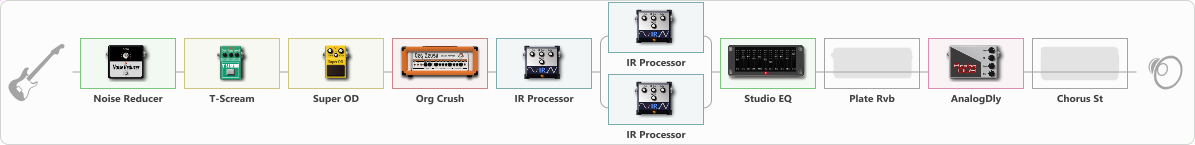# Metallica Kill 'Em All

Discussion in 'ToneLib-GFX presets' started by Tony the Metalhead, Dec 6, 2021.

1. ### Tony the MetalheadActive Member

Metallica Kill 'Em All

Preset name: Kill Em All

Updated

Effects chain:Effect: "Noise Reducer" (Dynamics / Filter), active - "yes"
{
"Sens" = 75
"Mode" = Hard
}

Effect: "T-Scream" (Overdrive / Distortion), active - "yes"
{
"Drive" = 5
"Tone" = 40
"Level" = 10
}

Effect: "Super OD" (Overdrive / Distortion), active - "yes"
{
"Drive" = 60
"Tone" = 40
"Level" = 40
}

Effect: "Org Crush" (Amp simulators), active - "yes"
{
"Gain" = 70
"Bass" = 20
"Middle" = 10
"Treble" = 40
"Presence" = 65
"Master" = 30
"Level (dB)" = 3
}

Effect: "IR Processor" (Cabinets), active - "yes"
{
"IR" = killem_LR_Stereo_IR
"Low Cut (Hz)" = 125
"Hi Cut (kHz)" = 20.0
"Mix" = 100
"Level (dB)" = 0
}

Effect: "Splitter" (Dynamics / Filter)
{
"A-Bypass" = Off
"A-Pan" = -25
"A-Level" = 55
"B-Bypass" = Off
"B-Pan" = 25
"B-Level" = 55

'A' branch:
{

Effect: "IR Processor" (Cabinets), active - "yes"
{
"IR" = OD-FB-GB-DYN-57-P10-30-R
"Low Cut (Hz)" = 0
"Hi Cut (kHz)" = 20.0
"Mix" = 100
"Level (dB)" = 0
}
}
'B' branch:
{

Effect: "IR Processor" (Cabinets), active - "yes"
{
"IR" = OD-FB-GB-DYN-57-P10-30-R
"Low Cut (Hz)" = 0
"Hi Cut (kHz)" = 20.0
"Mix" = 100
"Level (dB)" = 0
}
}
}

Effect: "Studio EQ" (Dynamics / Filter), active - "yes"
{
"31 Hz" = -7
"62 Hz" = -3
"125 Hz" = 3
"250 Hz" = -12
"500 Hz" = 1
"1 kHz" = -6
"2 kHz" = -6
"4 kHz" = -6
"8 kHz" = -6
"16 kHz" = 10
"Level (dB)" = 6
}

Effect: "Plate Rvb" (Reverberation), active - "no"
{
"Time" = 1.8
"PreDelay" = 5
"LoDamp" = 100
"HiDamp" = 100
"Mix" = 15
}

Effect: "AnalogDly" (Delay), active - "yes"
{
"Time" = 200
"Feedback" = 20
"Tone" = 50
"Mix" = 30
}

Effect: "Chorus St" (Modulation / Sfx), active - "no"
{
"Speed" = 1.8
"Depth" = 25
"Center" = 2.5
"Mix" = 25
}

Note: You will need to download and install the ToneLib-GFX software to use the preset.

#### Attached Files:

• ###### Metallica_Kill_Em_All.tlgfx
File size:
5.9 KB
Views:
2,812
qred, darex, TheAragones and 10 others like this.
2. Sorry for my bad english and my ignorance, but killem_L_IR appears in the IR Processor plugin. How do you get that impulse?
Thank you

rodrigo neves likes this.